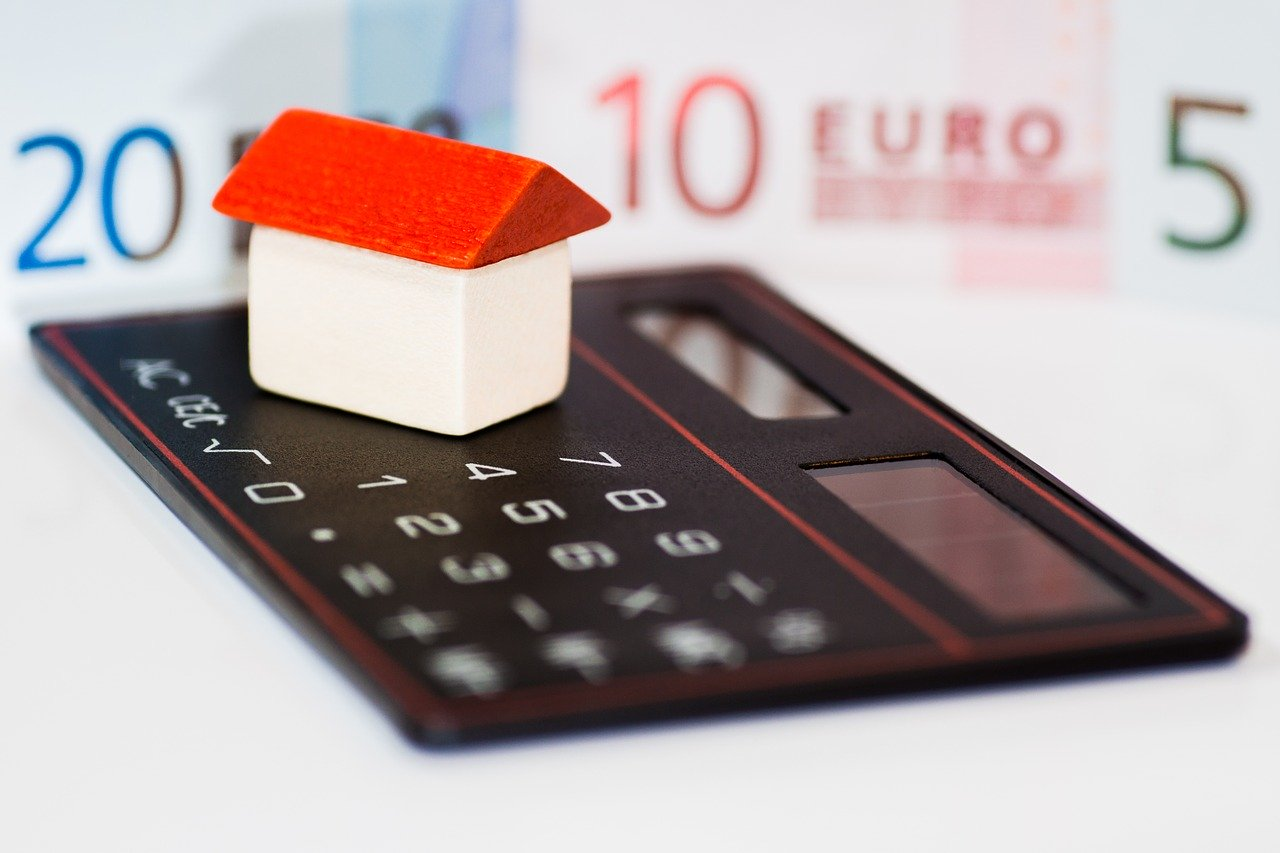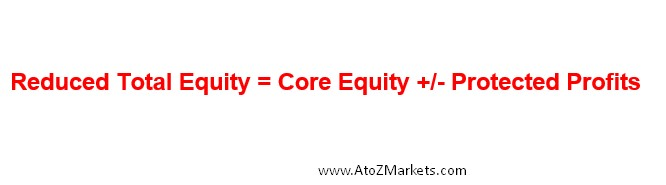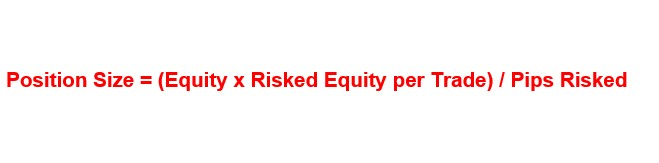# How to Calculate Position Size in ForexCalculating the position size in the forex is a vital part of money management. A trader should know the skills to minimize risks and maximize rewards. This article will guide you with some rules to calculate the position in the forex market.

Risk management is an important part of all traders. You should ask yourself how many lots you are going to buy before entering any trade. You should calculate position size according to your investment in any money management technique you follow.

## What is Position Size in Forex?

To identify the maximum risk size of trade, you should find the distance between your stop loss and your entry. Therefore, you should determine the pips and the lot size to calculate the ultimate risk in the dollar value. Risk per trade in currency value helps the trader to stay consistent with the maximum tolerable losses. Therefore, you have options to close your position when you see this going further towards the stop-loss levels.

To Calculate the Position Size in Forex, you need to know:

• Percentage of your investment you are willing to risk
• The distance of the entry price and the stop loss of your trade
• Pip value per a standard lot of a currency pairLike, if you have an account with 10,000 US dollars and you are ready to take 2% risk per trade. You are entering a position on the EURUSD and the stop loss for your trade is set at a distance of 100 pips. And the current pip value per standard lot is 1000 US Dollars. You are able to calculate the position’s size by using the formula:If you are going to open several positions, the same equation should be used for all of your open positions.

## Calculate the Position Size in Forex

If you open a trading account in a broker and open multiple positions with multiple trading sizes, you may face a problem with a risk of drawdown. In that case, calculating the position size may save you from any unexpected stop out.

However, you can determine the position size based on the balance on your account or based on a single trade. There are several ways to calculate the position size in forex- You need to choose the suitable one for you.

### Calculation Based on Total Equity

According to this model, the total equity is determined by the total available amount in the account balance and the value of all open positions. The value of the open position might be positive or negative. If you have open positions, the risk for a new position will be calculated with the balance plus the unrealized profits/ losses.If you have a balance of \$1000 in your trading account and a floating profit of \$200, the overall equity will be \$1200. In that case, if you want to take a 2% risk for your next trade, you should allow a maximum of \$24 (1200 X 2%= 24) for a single trade.

### Core Equity Model or Free Margin

It is another method for determining the position size based on the total balance. The core equity model is the difference between the account balance and the amount used for the open position. It is a simple model that takes into account the amount needed to open a position.For example, you have a balance of \$10,000 and you enter a trade with one mini lot (\$1,000), and then your core equity or free margin will reduce to \$9,000. After that, if you enter another \$1,000 trade, your core equity will be \$8000.

As your core equity rises, you can make a trade with a bigger lot size or smaller if your equity falls.  Following the example, if you open a second position, your core equity would fall to \$8,000. Therefore, you should limit your risk to \$800 to ensure the 10% available margin.

### Reduced Total Equity Model

The calculation of equity is the difference between the balance and the amount used for open positions. However, any benefit from a protecting stop loss should also be accounted for.We use the stop loss to minimize the risk in the Forex markets. The Forex position is a margin position. As a trader, you have an obligation to make good on losses, but there is not any physical delivery of the specified currency. You are not taking possessions of the following \$10000 as you trade a mini lot. Therefore, your position size in the forex should be based on this.

This is the formula for calculating a position’s size considering equity:### Risk of Specific Amount Per Trade

This model is very important to determine the position size in forex for a single trade; you can set the risk of percentage or dollar amount on each trade. Most professional traders risk a 2% risk or less for their accounts.

If you have a \$10,000 in trading account, you could risk \$100 (10,000 X 1%= 100) per trade. If your risk limit is 0.5%, you can risk \$50 (10,000 X 0.5%= 50) per trade. Moreover, you can also set a fixed dollar amount that may be equivalent to 1% or less.

Account risk should be constant while other trading variables change. Never take a 5% risk on a trade or 1% on the next, and 3% on another. You should choose your percentage or fixed amount and stick with it.

### Calculate the Position Size Based on Pip Risk on a Trade

Pip risk on each trade is the difference between the entry point and the stop loss level. It varies based on market volatility, probability or strategy. Sometimes a trade may have 100 pips of risk, and another trade may have 150 pips of risk. When you make a trade, identify your entry point and your stop-loss level.

You should consider the market context to determine the possible pips as a risk or use a gold price calculator if you trade gold. For the volatile market, the stop loss level will be higher than the nonvolatile market. Therefore, pip risk for a trade is not fixed for all trades.

If you take a trade with 0.50 lot with a stop loss of 50 pips, your risk per trade will be \$250. However, if you take the same trade with 100 pips stop loss, your position size in forex will reduce to 0.25 to keep the loss per trade unchanged.

### Calculate the Position Size Based on Pip Value for a Trade

If you are calculating the position size in forex for a currency pair where the U.S. dollar is the second currency and your trading account is funded with dollars, the pip values are fixed for different lot sizes. For a micro lot, the pip value for one pip is \$0.10, or 10 cents. For a mini lot, it is \$1 and for a standard lot, it is \$10. Therefore, you can easily determine the position size in forex with the price changes in pips. If your stop-loss is 100 pips while the allowable loss is 100\$, the lot size will be 0.10.

Moreover, if your trading account is funded with dollars while the quote currency is not the U.S. dollar, you need to multiply the pip values by the exchange rates.

Let us say you are trading the EURGBP pair, and the GBPUSD is trading at 1.2219. In that case, the micro lot of EUR/GBP, the pip value would be \$0.12 for one pip. For a mini lot \$1.22 and for a standard lot, it will be \$12.21. Therefore, if your allowable stop loss is 100 pips and allowable loss is \$100, the lot size should be 0.12.

## Conclusion

What other things a trader should consider?

Calculating the position size in the forex is a vital part of money management. However, there are other things to consider, like the market context. If you are trading a decrease period of a particular timeframe or you are trading in a volatile market, you should use a small lot size even if your position size allows taking a bigger lot.

Moreover, a determination of the position size in the forex will save you from various unexpected movements that may eat your total balance. There are some uncertainties in every market, so you should be cautious and stick to your money management rules.

How you calculate Position size while trading in Forex Market? Let us know in the comments section below.